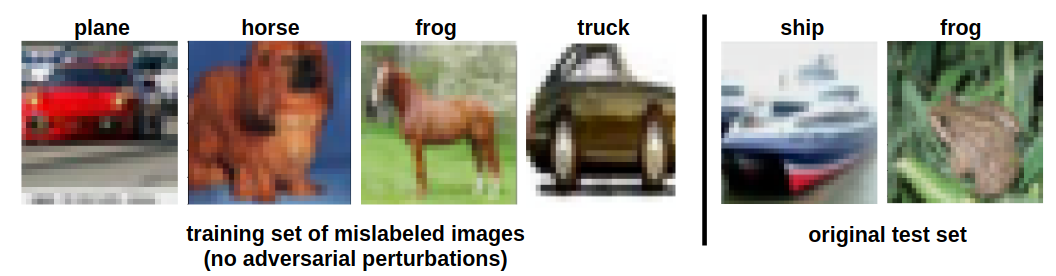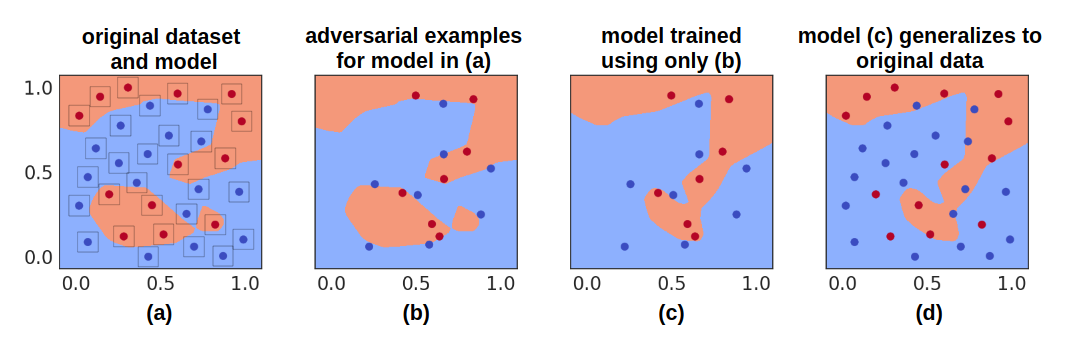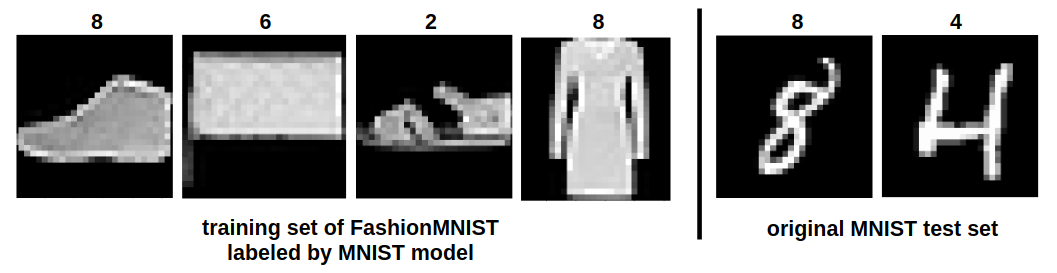# Learning from Incorrectly Labeled Data

Aug. 6, 2019

### DOI

10.23915/distill.00019.6

This article is part of a discussion of the Ilyas et al. paper “Adversarial examples are not bugs, they are features”. You can learn more in the main discussion article .

Other Comments Comment by Ilyas et al.

Section 3.2 of Ilyas et al. (2019) shows that training a model on only adversarial errors leads to non-trivial generalization on the original test set. We show that these experiments are a specific case of learning from errors. We start with a counterintuitive result — we take a completely mislabeled training set (without modifying the inputs) and use it to train a model that generalizes to the original test set. We then show that this result, and the results of Ilyas et al. (2019), are a special case of model distillation. In particular, since the incorrect labels are generated using a trained model, information about the trained model is being “leaked” into the dataset. We begin with the following question: what if we took the images in the training set (without any adversarial perturbations) and mislabeled them? Since the inputs are unmodified and mislabeled, intuition says that a model trained on this dataset should not generalize to the correctly-labeled test set. Nevertheless, we show that this intuition fails — a model can generalize. We first train a ResNet-18 on the CIFAR-10 training set for two epochs. The model reaches a training accuracy of 62.5% and a test accuracy of 63.1%. Next, we run the model on all of the 50,000 training data points and relabel them according to the model’s predictions. Then, we filter out all the correct predictions. We are now left with an incorrectly labeled training set of size 18,768. We show four examples on the left of the Figure below:1

We then randomly initialize a new ResNet-18 and train it only on this mislabeled dataset. We train for 50 epochs and reach an accuracy of 49.7% on the original test set. The new model has only ever seen incorrectly labeled, unperturbed images but can still non-trivially generalize.

## This is Model Distillation Using Incorrect Predictions

How can this model and the models in Ilyas et al. (2019) generalize without seeing any correctly labeled data? Here, we show that since the incorrect labels are generated using a trained model, information is being “leaked” about that trained model into the mislabeled examples. In particular, this an indirect form of model distillation — training on this dataset allows a new model to somewhat recover the features of the original model.

We first illustrate this distillation phenomenon using a two-dimensional problem. Then, we explore other peculiar forms of distillation for neural networks — -we transfer knowledge despite the inputs being from another task.

### Two-dimensional Illustration of Model Distillation

We construct a dataset of adversarial examples using a two-dimensional binary classification problem. We generate 32 random two-dimensional data points in $[0,1]^2$ and assign each point a random binary label. We then train a small feed-forward neural network on these examples, predicting 32/32 of the examples correctly (panel (a) in the Figure below).2

Next, we create adversarial examples for the original model using an $l_{\infty}$ ball of radius $\epsilon=0.12$. In panel (a) of the Figure above, we display the $\epsilon$-ball around each training point. In panel (b), we show the adversarial examples which cause the model to change its prediction (from correct to incorrect). We train a new feed-forward neural network on this dataset, resulting in the model in panel (c).

Although this new model has never seen a correctly labeled example, it is able to perform non-trivially on the original dataset, predicting $23/32$ of the inputs correctly (panel (d) in the Figure). The new model’s decision boundary loosely matches the original model’s decision boundary, i.e., the original model has been somewhat distilled after training on its adversarial examples. This two-dimensional problem presents an illustrative version of the intriguing result that distillation can be performed using incorrect predictions.

### Other Peculiar Forms of Distillation

Our experiments show that we can distill models using mislabeled examples. In what other peculiar ways can we learn about the original model? Can we use only out-of-domain data?

We train a simple CNN model on MNIST, reaching 99.1% accuracy. We then run this model on the FashionMNIST training set and save its argmax predictions. The resulting dataset is nonsensical to humans — a “dress” is labeled as an “8″.3

We then initialize a new CNN model and train it on this mislabeled FashionMNIST data. The resulting model reaches 91.04% accuracy on the MNIST test set. Furthermore, if we normalize the FashionMNIST images using the mean and variance statistics for MNIST, the model reaches 94.5% accuracy on the MNIST test set. This is another instance of recovering a functionally similar model to the original despite the new model only training on erroneous predictions.

### Summary

These results show that training a model using mislabeled adversarial examples is a special case of learning from prediction errors. In other words, the perturbations added to adversarial examples in Section 3.2 of Ilyas et al. (2019) are not necessary to enable learning.

To cite Ilyas et al.’s response, please cite their collection of responses.
Response Summary: Note that since our experiments work across different architectures, “distillation” in weight space does not occur. The only distillation that can arise is “feature space” distillation, which is actually exactly our hypothesis. In particular, feature-space distillation would not work in World 1 — if the adversarial examples we generated did not exploit useful features, we should not have been able to “distill” a useful model from them. (In fact, one might think of normal model training as just “feature distillation” of the humans that labeled the dataset.) Furthermore, the hypothesis that all we need is enough model-consistent points in order to recover a model, seems to be disproven by Preetum’s “bugs-only dataset” and other (e.g. ) settings.

Response: Since our experiments work across different architectures, “distillation” in weight space cannot arise. Thus, from what we understand, the “distillation” hypothesis suggested here is referring to “feature distillation” (i.e. getting models which use the same features as the original), which is actually precisely our hypothesis too. Notably, this feature distillation would not be possible if adversarial examples did not rely on “flipping” features that are good for classification (see World 1 and World 2) — in that case, the distilled model would only use features that generalize poorly, and would thus generalize poorly itself.

Moreover, we would argue that in the experiments presented (learning from mislabeled data), the same kind of distillation is happening. For instance, a moderately accurate model might associate “green background” with “frog” thus labeling “green” images as “frogs” (e.g., the horse in the comment’s figure). Training a new model on this dataset will thus associate “green” with “frog” achieving non-trivial accuracy on the test set (similarly for the “learning MNIST from Fashion-MNIST” experiment in the comment). This corresponds exactly to learning features from labels, akin to how deep networks “distill” a good decision boundary from human annotators. In fact, we find these experiments a very interesting illustration of feature distillation that complements our findings.

We also note that an analogy to logistic regression here is only possible due to the low VC-dimension of linear classifiers (namely, these classifiers have dimension $d$). In particular, given any classifier with VC-dimension $k$, we need at least $k$ points to fully specify the classifier. Conversely, neural networks have been shown to have extremely large VC-dimension (in particular, bigger than the size of the training set ). So even though labelling $d+1$ random points model-consistently is sufficient to recover a linear model, it is not necessarily sufficient to recover a deep neural network. For instance, Milli et al. are not able to reconstruct a ResNet-18 using only its predictions on random Gaussian inputs. (Note that we are using a ResNet-50 in our experiments.)

Finally, it seems that the only potentially problematic explanation for our experiments (namely, that enough model-consistent points can recover a classifier) is disproved by Preetum’s experiment. In particular, Preetum is able to design a dataset where training on mislabeled inputs that are model-consistent does not at all recover the decision boundary of the original model. More generally, the “model distillation” perspective raised here is unable to distinguish between the dataset created by Preetum below, and those created with standard PGD (as in our $\widehat{\mathcal{D}}_{det}$ and $\widehat{\mathcal{D}}_{rand}$ datasets).

You can find more responses in the main discussion article.
1. Distilling the Knowledge in a Neural Network[PDF]
Hinton, G., Vinyals, O. and Dean, J., 2015.
2. Model reconstruction from model explanations
Milli, S., Schmidt, L., Dragan, A.D. and Hardt, M., 2018. arXiv preprint arXiv:1807.05185.
3. Understanding deep learning requires rethinking generalization
Zhang, C., Bengio, S., Hardt, M., Recht, B. and Vinyals, O., 2016. International Conference on Learning Representations (ICLR).